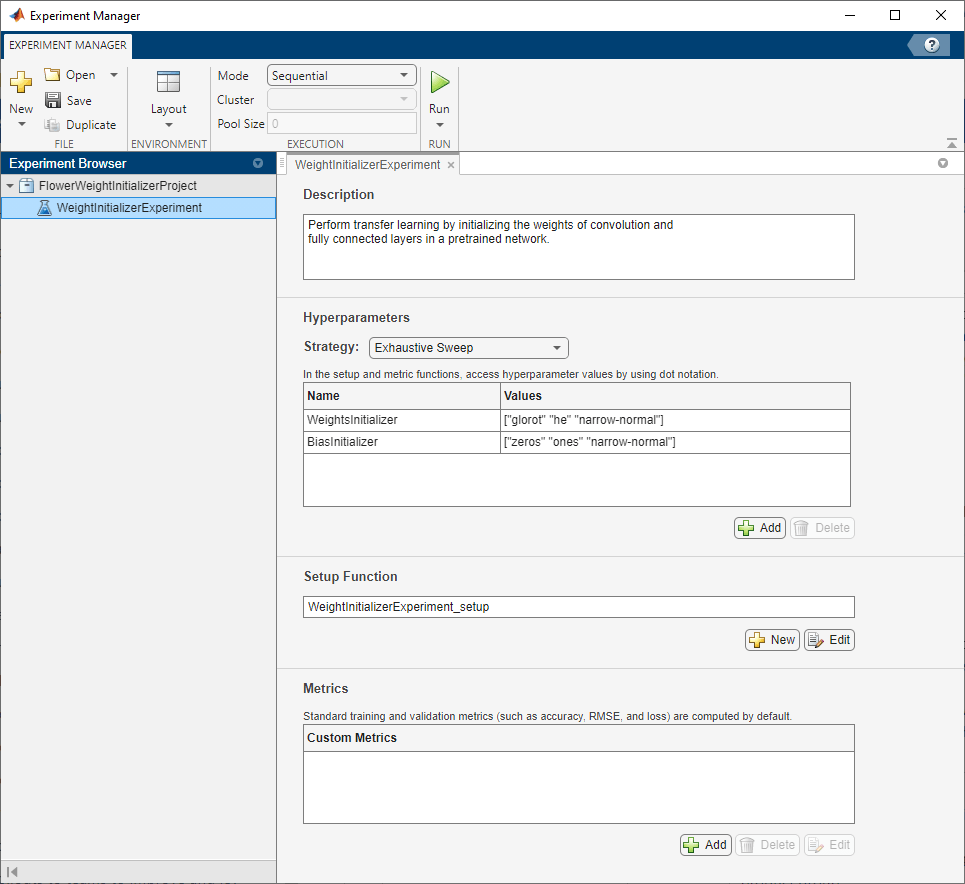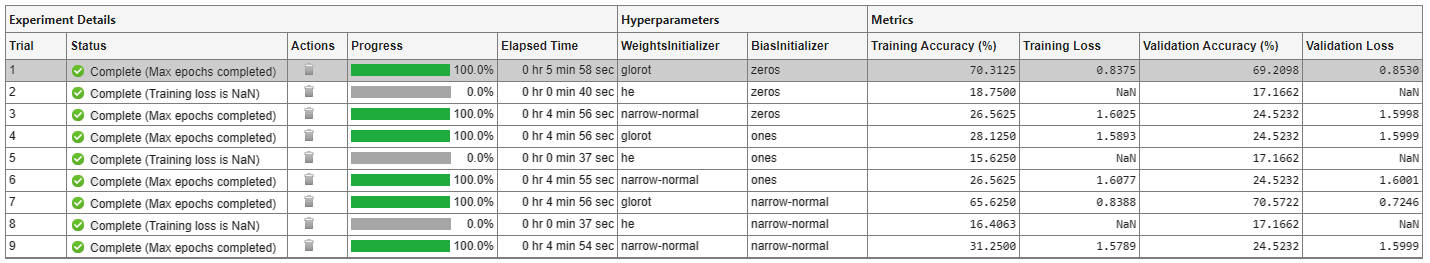# Experiment with Weight Initializers for Transfer Learning

Since R2020a

This example shows how to configure an experiment that initializes the weights of convolution and fully connected layers using different weight initializers for training. To compare the performance of different weight initializers for your task, create an experiment using this example as a guide.

When training a deep learning network, the initialization of layer weights and biases can have a big impact on how well the network trains. The choice of initializer has a bigger impact on networks without batch normalization layers. For more information on weight initializers, see Compare Layer Weight Initializers.

### Open Experiment

First, open the example. Experiment Manager loads a project with a preconfigured experiment that you can inspect and run. To open the experiment, in the Experiment Browser pane, double-click WeightInitializerExperiment.Built-in training experiments consist of a description, a table of hyperparameters, a setup function, and a collection of metric functions to evaluate the results of the experiment. For more information, see Configure Built-In Training Experiment.

The Description field contains a textual description of the experiment. For this example, the description is:

```Perform transfer learning by initializing the weights of convolution and fully connected layers in a pretrained network.```

The Hyperparameters section specifies the strategy and hyperparameter values to use for the experiment. When you run the experiment, Experiment Manager trains the network using every combination of hyperparameter values specified in the hyperparameter table. This example uses the hyperparameters `WeightsInitializer` and `BiasInitializer` to specify the weight and bias initializers for the convolution and fully connected layers in a pretrained network. For more information about these initializers, see `WeightsInitializer` and `BiasInitializer`.

The Setup Function section specifies a function that configures the training data, network architecture, and training options for the experiment. To open this function in MATLAB® Editor, click Edit. The code for the function also appears in Setup Function. The input to the setup function is a structure with fields from the hyperparameter table. The function returns three outputs that you use to train a network for image classification problems. In this example, the setup function:

```lgraph = googlenet(Weights="none"); ```

```url = "http://download.tensorflow.org/example_images/flower_photos.tgz"; downloadFolder = tempdir; filename = fullfile(downloadFolder,"flower_dataset.tgz"); imageFolder = fullfile(downloadFolder,"flower_photos"); if ~exist(imageFolder,"dir") disp("Downloading Flower Dataset (218 MB)...") websave(filename,url); untar(filename,downloadFolder) end imds = imageDatastore(imageFolder, ... IncludeSubfolders=true, ... LabelSource="foldernames"); [imdsTrain,imdsValidation] = splitEachLabel(imds,0.9); inputSize = lgraph.Layers(1).InputSize; augimdsTrain = augmentedImageDatastore(inputSize,imdsTrain); augimdsValidation = augmentedImageDatastore(inputSize,imdsValidation); ```
• Initializes the input weight in the convolution and fully connected layers by using the initializers specified in the hyperparameter table. The helper function `findLayersToReplace` determines the layers in the network architecture that can be modified for transfer learning. To view the code for this function, see Find Layers to Replace.

```numClasses = numel(categories(imdsTrain.Labels)); weightsInitializer = params.WeightsInitializer; biasInitializer = params.BiasInitializer; learnableLayer = findLayersToReplace(lgraph); newLearnableLayer = fullyConnectedLayer(numClasses,Name="new_fc"); lgraph = replaceLayer(lgraph,learnableLayer.Name,newLearnableLayer); for i = 1:numel(lgraph.Layers) layer = lgraph.Layers(i); if class(layer) == "nnet.cnn.layer.Convolution2DLayer" || ... class(layer) == "nnet.cnn.layer.FullyConnectedLayer" layerName = layer.Name; newLayer = layer; newLayer.WeightsInitializer = weightsInitializer; newLayer.BiasInitializer = biasInitializer; lgraph = replaceLayer(lgraph,layerName,newLayer); end end ```
• Defines a `trainingOptions` object for the experiment. The example trains the network for 10 epochs, using a mini-batch size of 128 and validating the network every 5 epochs.

```miniBatchSize = 128; validationFrequencyEpochs = 5; numObservations = augimdsTrain.NumObservations; numIterationsPerEpoch = floor(numObservations/miniBatchSize); validationFrequency = validationFrequencyEpochs * numIterationsPerEpoch; options = trainingOptions("sgdm", ... MaxEpochs=10, ... MiniBatchSize=miniBatchSize, ... Shuffle="every-epoch", ... ValidationData=augimdsValidation, ... ValidationFrequency=validationFrequency, ... Verbose=false); ```

The Metrics section specifies optional functions that evaluate the results of the experiment. This example does not include any custom metric functions.

### Run Experiment

When you run the experiment, Experiment Manager trains the network defined by the setup function multiple times. Each trial uses a different combination of hyperparameter values. By default, Experiment Manager runs one trial at a time. If you have Parallel Computing Toolbox™, you can run multiple trials at the same time or offload your experiment as a batch job in a cluster:

• To run one trial of the experiment at a time, on the Experiment Manager toolstrip, set Mode to `Sequential` and click Run.

• To run multiple trials at the same time, set Mode to `Simultaneous` and click Run. If there is no current parallel pool, Experiment Manager starts one using the default cluster profile. Experiment Manager then runs as many simultaneous trials as there are workers in your parallel pool. For best results, before you run your experiment, start a parallel pool with as many workers as GPUs. For more information, see Use Experiment Manager to Train Networks in Parallel and GPU Computing Requirements (Parallel Computing Toolbox).

• To offload the experiment as a batch job, set Mode to `Batch Sequential` or `Batch Simultaneous`, specify your cluster and pool size, and click Run. For more information, see Offload Deep Learning Experiments as Batch Jobs to a Cluster.

A table of results displays the accuracy and loss for each trial.Note that, for the trials that use the He weight initializer, Experiment Manager interrupts the training because the training and validation loss become undefined after a few iterations. Continuing the training for those trials does not produce any useful results. In the results table, the Status column indicates the reason for stopping these trials (`Training loss is NaN`).

When the experiment finishes, you can sort the results table by column, filter trials by using the Filters pane, or record observations by adding annotations. For more information, see Sort, Filter, and Annotate Experiment Results.

To test the performance of an individual trial, export the trained network or the training information for the trial. On the Experiment Manager toolstrip, select Export > Trained Network or Export > Training Information, respectively. For more information, see net and info. To save the contents of the results table as a nested `table` array in the MATLAB workspace, select Export > Results Table.

### Close Experiment

In the Experiment Browser pane, right-click FlowerWeightInitializerProject and select Close Project. Experiment Manager closes the experiment and results contained in the project.

### Setup Function

This function configures the training data, network architecture, and training options for the experiment. The input to this function is a structure with fields from the hyperparameter table. The function returns three outputs that you use to train a network for image classification problems.

```function [augimdsTrain,lgraph,options] = WeightInitializerExperiment_setup(params) ```

```lgraph = googlenet(Weights="none"); ```

```url = "http://download.tensorflow.org/example_images/flower_photos.tgz"; downloadFolder = tempdir; filename = fullfile(downloadFolder,"flower_dataset.tgz"); imageFolder = fullfile(downloadFolder,"flower_photos"); if ~exist(imageFolder,"dir") disp("Downloading Flower Dataset (218 MB)...") websave(filename,url); untar(filename,downloadFolder) end imds = imageDatastore(imageFolder, ... IncludeSubfolders=true, ... LabelSource="foldernames"); [imdsTrain,imdsValidation] = splitEachLabel(imds,0.9); inputSize = lgraph.Layers(1).InputSize; augimdsTrain = augmentedImageDatastore(inputSize,imdsTrain); augimdsValidation = augmentedImageDatastore(inputSize,imdsValidation); ```

Define Network Architecture

```numClasses = numel(categories(imdsTrain.Labels)); weightsInitializer = params.WeightsInitializer; biasInitializer = params.BiasInitializer; learnableLayer = findLayersToReplace(lgraph); newLearnableLayer = fullyConnectedLayer(numClasses,Name="new_fc"); lgraph = replaceLayer(lgraph,learnableLayer.Name,newLearnableLayer); for i = 1:numel(lgraph.Layers) layer = lgraph.Layers(i); if class(layer) == "nnet.cnn.layer.Convolution2DLayer" || ... class(layer) == "nnet.cnn.layer.FullyConnectedLayer" layerName = layer.Name; newLayer = layer; newLayer.WeightsInitializer = weightsInitializer; newLayer.BiasInitializer = biasInitializer; lgraph = replaceLayer(lgraph,layerName,newLayer); end end ```

Specify Training Options

```miniBatchSize = 128; validationFrequencyEpochs = 5; numObservations = augimdsTrain.NumObservations; numIterationsPerEpoch = floor(numObservations/miniBatchSize); validationFrequency = validationFrequencyEpochs * numIterationsPerEpoch; options = trainingOptions("sgdm", ... MaxEpochs=10, ... MiniBatchSize=miniBatchSize, ... Shuffle="every-epoch", ... ValidationData=augimdsValidation, ... ValidationFrequency=validationFrequency, ... Verbose=false); ```
```end ```

### Find Layers to Replace

This function finds the single classification layer and the preceding learnable (fully connected or convolutional) layer of the layer graph `lgraph`.

```function [learnableLayer,classLayer] = findLayersToReplace(lgraph) if ~isa(lgraph,"nnet.cnn.LayerGraph") error("Argument must be a LayerGraph object.") end src = string(lgraph.Connections.Source); dst = string(lgraph.Connections.Destination); layerNames = string({lgraph.Layers.Name}'); isClassificationLayer = arrayfun(@(l) ... (isa(l,"nnet.cnn.layer.ClassificationOutputLayer")|isa(l,"nnet.layer.ClassificationLayer")), ... lgraph.Layers); if sum(isClassificationLayer) ~= 1 error("Layer graph must have a single classification layer.") end classLayer = lgraph.Layers(isClassificationLayer); currentLayerIdx = find(isClassificationLayer); while true if numel(currentLayerIdx) ~= 1 error("Layer graph must have a single learnable layer preceding the classification layer.") end currentLayerType = class(lgraph.Layers(currentLayerIdx)); isLearnableLayer = ismember(currentLayerType, ... ["nnet.cnn.layer.FullyConnectedLayer","nnet.cnn.layer.Convolution2DLayer"]); if isLearnableLayer learnableLayer = lgraph.Layers(currentLayerIdx); return end currentDstIdx = find(layerNames(currentLayerIdx) == dst); currentLayerIdx = find(src(currentDstIdx) == layerNames); end end ```